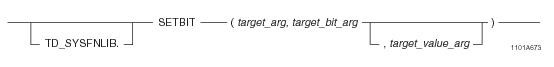## SQL Functions, Operators, Expressions, and Predicates

Product
Release Number
15.00
Language
English (United States)
Last Update
2018-09-24
dita:id
B035-1145
lifecycle
previous
Product Category

## SETBIT

### Purpose

Sets the value of the bit specified by target_bit_arg to the value of target_value_arg in the target_arg byte expression.

### Syntaxwhere:

 Syntax element… Specifies… TD_SYSFNLIB the name of the database where the function is located. target_arg a numeric or variable byte expression. target_bit_arg an integer expression. target_value_arg an optional integer value. Only a value of 0 or 1 is allowed. If target_value_arg is not specified, the default is 1.

### ANSI Compliance

This is a Teradata extension to the ANSI SQL:2011 standard.

### Description

SETBIT takes the target_arg input and sets the bit specified by target_bit_arg to the value, 0 or 1, as provided by the target_value_arg argument.

The target_value_arg parameter only accepts a value of 0 or 1. If a value for target_value_arg is not specified, the default value of 1 is used.

The range of input values for target_bit_arg can vary from 0 (bit 0 is the least significant bit) to the (sizeof(target_arg) - 1).

If target_bit_arg is negative or out-of-range (meaning that it exceeds the size of target_arg), an error is returned.

If any of the input arguments is NULL, the function returns NULL.

### Argument Types and Rules

SETBIT is an overloaded scalar function. It is defined with the following parameter data types for the following (target_arg, target_bit_arg[,target_value_arg]) input combinations:

 target_arg type target_bit_arg type target_value_arg type (optional) BYTEINT INTEGER INTEGER SMALLINT INTEGER INTEGER INTEGER INTEGER INTEGER BIGINT INTEGER INTEGER VARBYTE(n) INTEGER INTEGER

The maximum supported size (n) for VARBYTE is 8192 bytes.

All expressions passed to this function must either match these declared data types or can be converted to these types using the implicit data type conversion rules that apply to UDFs.

Note: The UDF implicit type conversion rules are more restrictive than the implicit type conversion rules normally used by Teradata Database. If any argument cannot be converted to one of the declared data types by following UDF implicit conversion rules, it must be explicitly cast. For details, see “Compatible Types” and “Parameter Types in Overloaded Functions” in SQL External Routine Programming.

If any argument cannot be converted to one of the declared data types, an error is returned indicating that no function exists that matches the DML UDF expression submitted.

### Result Type and Attributes

The result data type depends on the data type of the target_arg input argument that is passed to the function as shown in the following table:

 IF the data type of target_arg is... THEN the result type is... AND the result format is the default format for... BYTEINT BYTEINT BYTEINT SMALLINT SMALLINT SMALLINT INTEGER INTEGER INTEGER BIGINT BIGINT BIGINT VARBYTE(n) VARBYTE(n) VARBYTE(n)

The maximum supported size (n) for VARBYTE is 8192 bytes.

The default title for SETBIT is: SETBIT(target_arg, target_bit_arg[,target_value_arg]).

For information on default data type formats, see SQL Data Types and Literals.

### Example

The following query takes the input argument 23, which has a data type of BYTEINT and a binary representation of 00010111, and sets the value of the third bit to 1. The query result is a BYTEINT value of 23 or binary 00010111.

`   SELECT SETBIT(23,2);`

### Example

The following query takes the input argument 23, which has a data type of BYTEINT and a binary representation of 00010111, and sets the value of the third bit to 0. The query result is a BYTEINT value of 19 or binary 00010011.

`   SELECT SETBIT(23,2,0);`